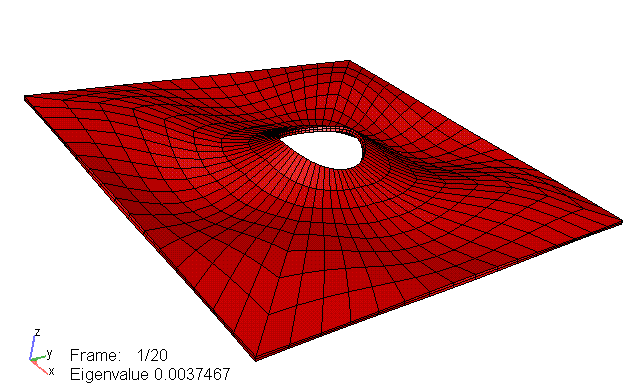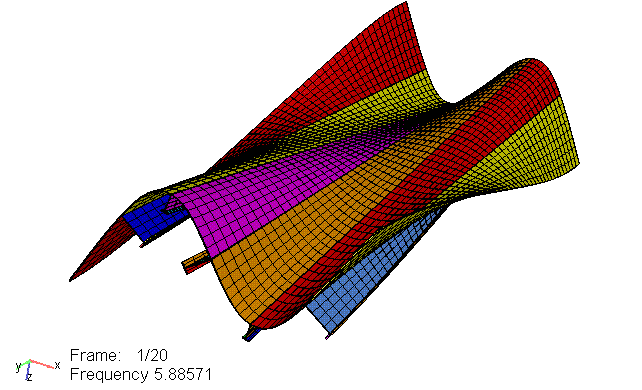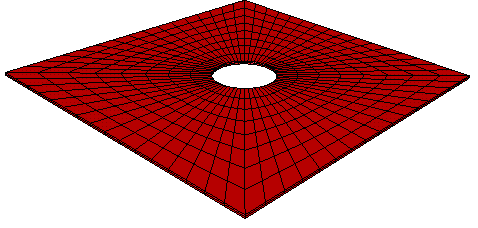###Isogeometric Analysis

Results
Project results will be published here.

Here are some preliminary results obtained with the prototype version of the iso-geometric simulation module developed in the ICADA project.

The prototype can do linear-elastic problems, static loads, linearized buckling and free vibration eigenvalue analysis.

## Linearized buckling analysis with NURBS

Below the fourth buckling mode for a simply supported square plate with a center hole is shown. The analysis is performed using 2nd order NURBS as basis functions.## Free vibration analysis of stiffened plate

Below the 11th vibration mode is shown, computed using 3rd order splines as basis functions.Published October 30, 2007